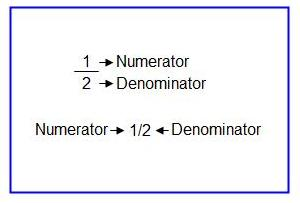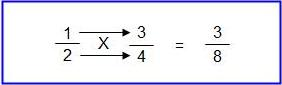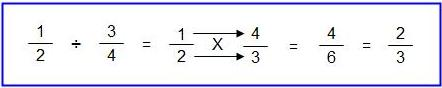How To Work With Fractions

Every home handyman has faced the problem of adding, subtracting, multiplying or dividing fractions. If you do not use fractions often, the task of working with them can be confusing.Figure 1 - Fractions

The following information should aid the home handyman in using fractions successfully.

Fraction Structure:

Fractions can be written in two different manners, but in either case all fractions are made up of two parts, the numerator and the denominator, as shown in Figure 1.

When you are faced with the problem of adding or subtracting fractions, you must have a common denominator.

The common denominator is the highest number:

As an example:

You need to calculate the result for the following problem; 1/2 − 2/3 + 3/4 − 5/6 + 7/12

The common denominator would be 12, which is the highest number. This means that you must express each fraction so that the denominator is 12 ( ×/12 ).

Work with each fraction independently using this formula:

Common Denominator / Denominator Of Fraction Being Converted X Numerator Of Fraction Being Converted.

 12 / 2 × 1 = 6 × 1 = 6 1/2 = 6/12 12 / 3 × 2 = 4 × 2 = 8 2/3 = 8/12 12 / 4 × 3 = 3 × 3 = 9 3/4 = 9/12 12 / 6 × 5 = 2 × 5 = 10 5/6 = 10/12 7/12 remains as is

You can now write the problem in a manner that can be easily dealt with:

6/12 − 8/12 + 9/12 − 10/12 + 7/12

As all of the denominators are the same it is only necessary to deal with the numerators.

6 − 8 + 9 − 10 + 7 = 4

The answer is 4/12 which in its lowest form is 1/3.

Multiplying Fractions:

Multiplying fractions is quite simple. Unlike addition or subtraction there is no need to convert the fractions to a common denominator.

Multiply the numerators. Then multiply the denominators. The result of the two multiplications is the answer.Dividing Fractions:

Dividing fractions is a little more complex than multiplying fractions. The easiest way to divide fractions is to take the reciprocal (reverse the numbers) of one of the fractions and multiply.

As an example:

You need to divide 1/2 by 3/4.

The reciprocal of 3/4 is 4/3 (the numbers are reversed).

1/2 divided by 3/4 is the same as 1/2 multiplied by 4/3.